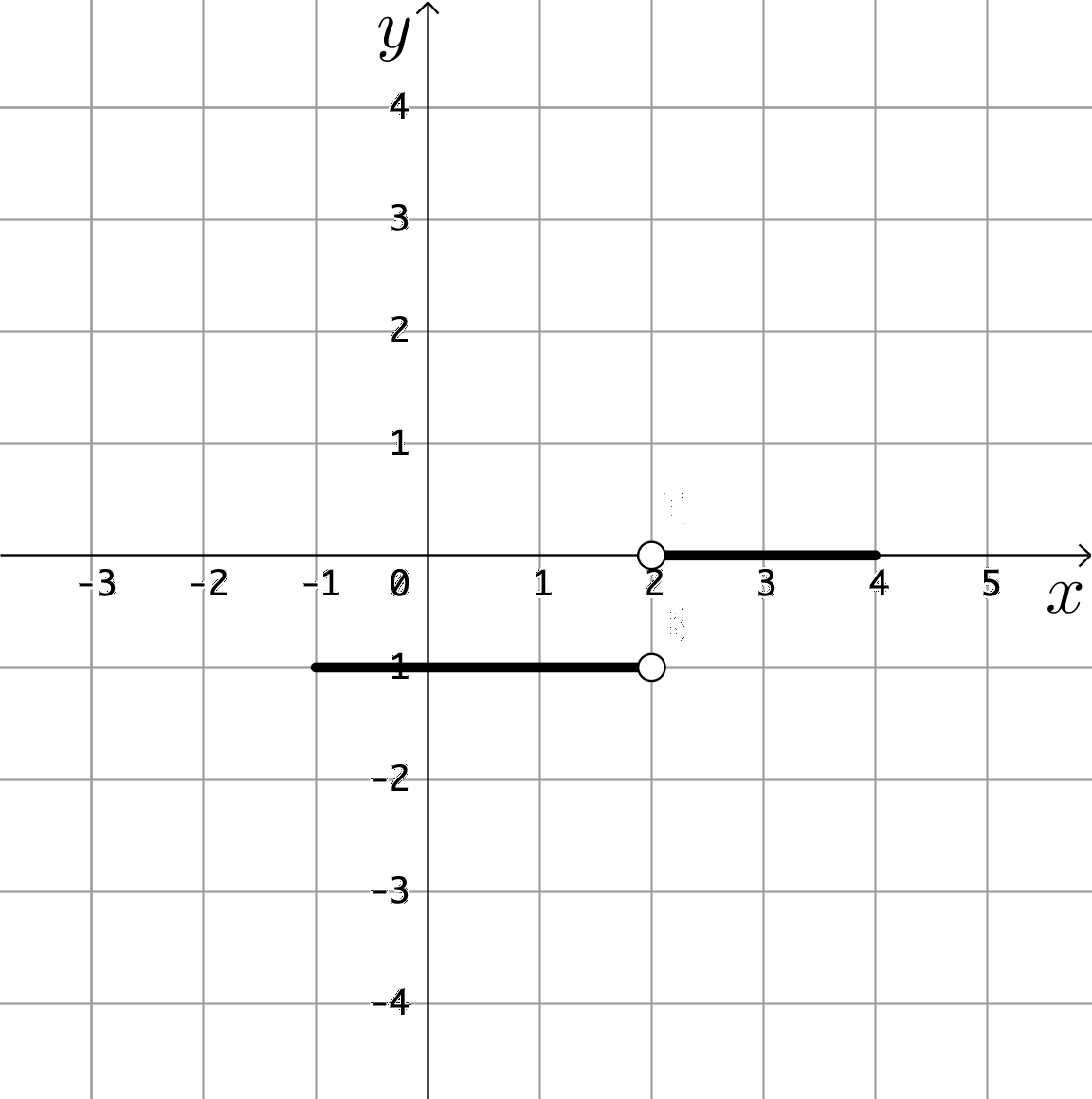$\newenvironment {prompt}{}{} \newcommand {\ungraded }{} \newcommand {\HyperFirstAtBeginDocument }{\AtBeginDocument }$

Determine an antiderivative for $f(x)=\sin (x^2)$
$2x \cos (x^2)$ $-\cos \left ( \frac {x^3}{3} \right )$ $\int _0^x \sin (t^2) dt$ $\int _0^x 2t \cos (t^2) dt$ $\int _0^x -\cos \left ( \frac {t^3}{3} \right ) dt$ $f(x)$ doesn’t have an antiderivative
If $F(x) = \int _0^x e^{2t} dt$, what is $F’(x)$?
$2e^{2x}$ $e^{2x}$ $\dfrac {e^{2x}}{2}$ $\dfrac {e^{2x+1}}{2x+1}$ $\dfrac {e^{2x}}{2}-\dfrac {1}{2}$ $\dfrac {e^{2x+1}}{2x+1}-e$ $\int _0^x 2e^{2t} dt$
$F(x) = \int _0^x 3t-9 dt$. On the interval $[0,3]$, $F(x)$ is:
The following graph is of $y = f(t)$ over the interval $[-1,4]$. Which of the following choices could be the corresponding graph of $F(x) = \int _{-1}^x f(t) dt$:
 Graph aGraph bGraph cGraph dGraph eGraph fGraph gGraph h